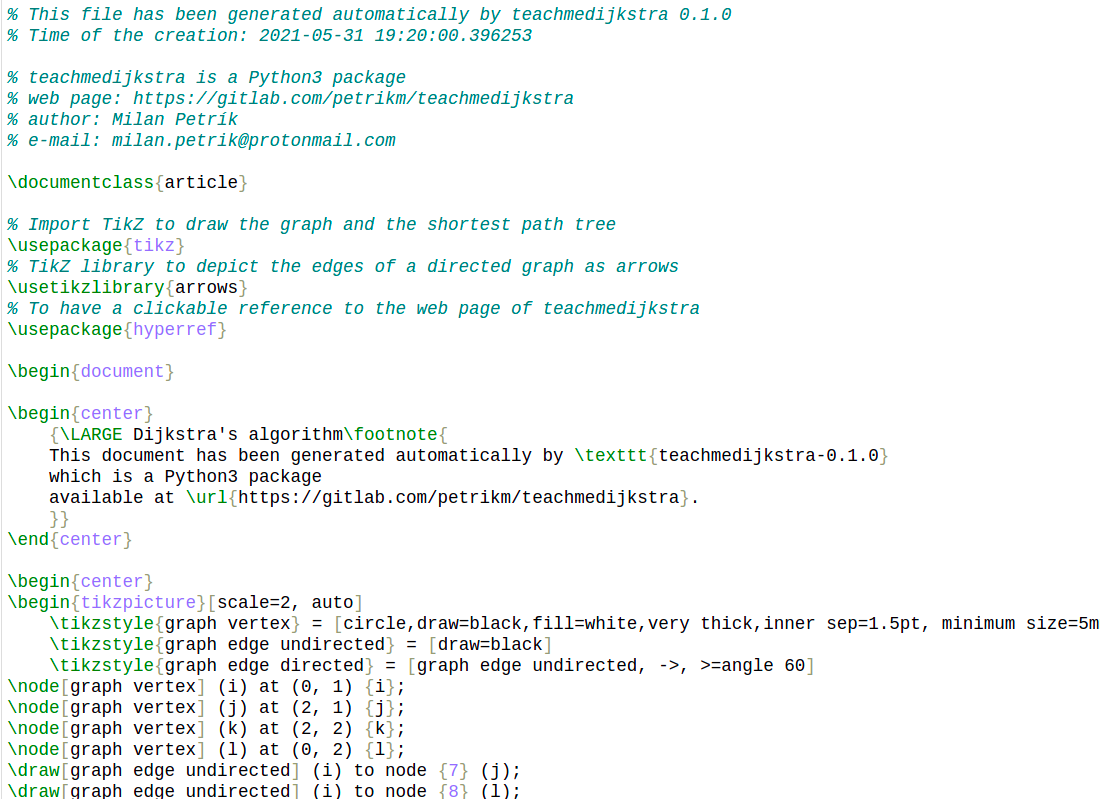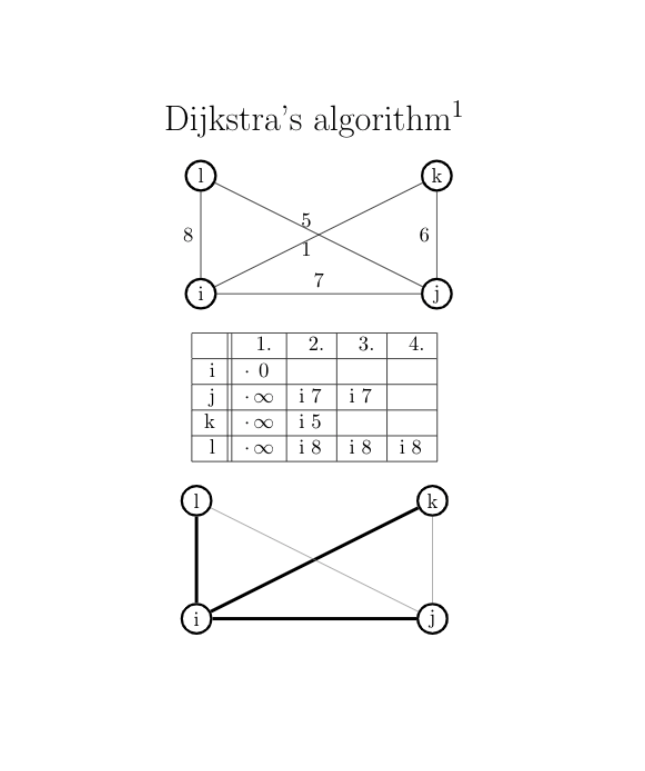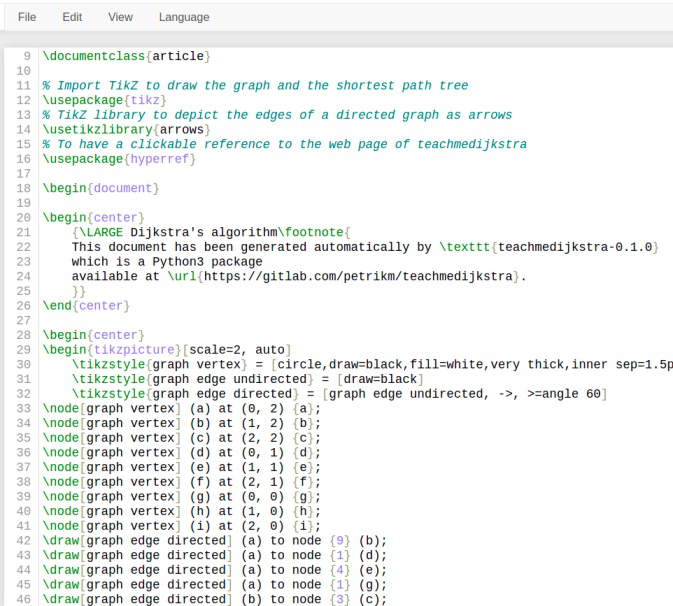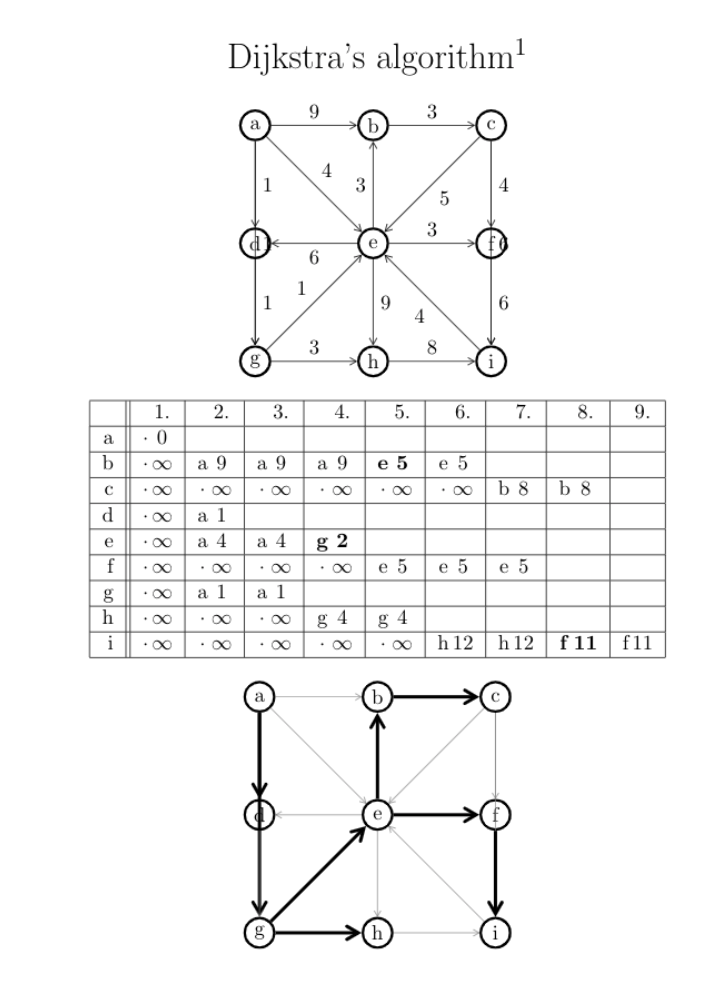Open in App
Not now

# teachmedijkstra library in Python

• Last Updated : 06 Jun, 2021

Dijkstra’s algorithm is very similar to Prim’s algorithm for minimum spanning trees. Like Prim’s MST, we generate a SPT (shortest path tree) with a given source as root. We maintain two sets, one set contains vertices included in the shortest path tree, other set includes vertices not yet included in the shortest path tree. At every step of the algorithm, we find a vertex that is in the other set (set of not yet included) and has a minimum distance from the source.

teachmedijkstra: Utility library helping in rendering explained Dijkstra’s alogirithm in latex format for teaching purposes. The rows of the matrix represent vertex of graph and column represent each unit time step of explanation, gets the shortest path tree constructed for shortest distance algorithm.

### Installation

This module does not come built-in with Python. To install it type the below command in the terminal.

`pip install teachmedijkstra`

After installation of the library, steps to proceed with are :

• Initializing the Undirected/Directed Graph
• Addition of Vertex and Edges to Graph
• Using Dijaskstra(), to input created graph with a starting point.
• run(), runs the algorithm.
• At the end, the latex file produced can be saved by the required name.

### Function Used :

• teachmedijkstra.UndirectedGraph() : Initializes the graph with undirected Graph.
• graph.addVertex(name, coordinate) : Adds name of vertex along with graphical coordinate it should belong to.
• graph.addEdge(strt, end, weight) : Adds edge from 1 node to another with particular weight.
• teachmedijkstra.Dijkstra(graph_obj, strt_point) : Initializes the variable with graph instance with starting point.
• dijkstra.run() : Runs the dijkstra algorithm.
• dijkstra.saveToLaTeXFile(file_name) : Saves the constructed graph in file_name in latex format.

Example 1:

## Python3

 `import` `teachmedijkstra ` ` `  `# getting graph ` `graph ``=` `teachmedijkstra.UndirectedGraph() ` ` `  `# initializing vertices ` `graph.addVertex(``"i"``, (``0``, ``1``)) ` `graph.addVertex(``"j"``, (``2``, ``1``)) ` `graph.addVertex(``"k"``, (``2``, ``2``)) ` `graph.addVertex(``"l"``, (``0``, ``2``)) ` ` `  `# initializing edges ` `graph.addEdge(``"i"``, ``"j"``, ``7``) ` `graph.addEdge(``"i"``, ``"l"``, ``8``) ` `graph.addEdge(``"j"``, ``"k"``, ``6``) ` `graph.addEdge(``"l"``, ``"j"``, ``1``) ` `graph.addEdge(``"k"``, ``"i"``, ``5``) ` ` `  ` `  `# creating graph from i. ` `dijkstra ``=` `teachmedijkstra.Dijkstra(graph, ``"i"``) ` `dijkstra.run() ` ` `  `# saving file ` `dijkstra.saveToLaTeXFile(``"undirectedDij.tex"``) `

Produced Latex File :Output ( After converting Latex ):Example 2: Directed Graph

In this example, we will create a directed graph with this module.

## Python3

 `import` `teachmedijkstra ` ` `  `# initializing Directed graph ` `graph ``=` `teachmedijkstra.DirectedGraph() ` ` `  `# initializing vertices ` `graph.addVertex(``"a"``, (``0``, ``2``)) ` `graph.addVertex(``"b"``, (``1``, ``2``)) ` `graph.addVertex(``"c"``, (``2``, ``2``)) ` `graph.addVertex(``"d"``, (``0``, ``1``)) ` `graph.addVertex(``"e"``, (``1``, ``1``)) ` `graph.addVertex(``"f"``, (``2``, ``1``)) ` `graph.addVertex(``"g"``, (``0``, ``0``)) ` `graph.addVertex(``"h"``, (``1``, ``0``)) ` `graph.addVertex(``"i"``, (``2``, ``0``)) ` ` `  `# adding edges ` `graph.addEdge(``"a"``, ``"b"``, ``9``) ` `graph.addEdge(``"b"``, ``"c"``, ``3``) ` `graph.addEdge(``"c"``, ``"f"``, ``4``) ` `graph.addEdge(``"e"``, ``"f"``, ``3``) ` `graph.addEdge(``"e"``, ``"d"``, ``6``) ` `graph.addEdge(``"d"``, ``"g"``, ``1``) ` `graph.addEdge(``"g"``, ``"h"``, ``3``) ` `graph.addEdge(``"h"``, ``"i"``, ``8``) ` `graph.addEdge(``"a"``, ``"d"``, ``1``) ` `graph.addEdge(``"e"``, ``"b"``, ``3``) ` `graph.addEdge(``"e"``, ``"h"``, ``9``) ` `graph.addEdge(``"f"``, ``"i"``, ``6``) ` `graph.addEdge(``"a"``, ``"e"``, ``4``) ` `graph.addEdge(``"c"``, ``"e"``, ``5``) ` `graph.addEdge(``"g"``, ``"e"``, ``1``) ` `graph.addEdge(``"i"``, ``"e"``, ``4``) ` `graph.addEdge(``"c"``, ``"i"``, ``6``) ` `graph.addEdge(``"a"``, ``"g"``, ``1``) ` ` `  ` `  `# calling Dijkstra fnc to perform algo. ` `dijkstra ``=` `teachmedijkstra.Dijkstra(graph, ``"a"``) ` `dijkstra.run() ` ` `  ` `  `# saving file ` `dijkstra.saveToLaTeXFile(``"directedDij.tex"``) `

Latex Output :Output :My Personal Notes arrow_drop_up
Related Articles